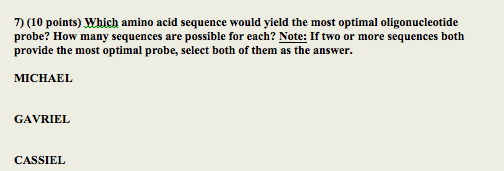Homework Help Question & Answers

Which amino acid sequence would yield the most optimal oligonucleotide probe? How many sequences ...

Which amino acid sequence would yield the most optimal oligonucleotide probe? How many sequences are possible for each?7) (10 points) Which amino acid sequence would yield the most optimal oligonucleotide probe? How many sequences are possible for each? Note: If two or more sequences both provide the most optimal probe, select both of them as the answer MICHAEL GAVRIEL CASSIEL

Ala-Met-Ser-Leu-Pro-Trp is having a total of 576 sequences.(4*1*6*6*4*1)

Gly-Trp-Asp-Met-His-Lys is having a total of 32 sequences.(4*1*2*1*2*2)

Arg-Ser-Met-Leu-Gln-Asn is having a total of 1864 sequences(6*6*1*6*2*2)

Cys-Val-Trp-Asn-Lys-Ile is having a total of 96 Sequences.(2*4*1*2*2*3)

From this the Arg-Ser-Met-Leu-Gln-Asn have the most optimal oligonucleotide probe with 1864 sequences and the second one is  Ala-Met-Ser-Leu-Pro-Trp with 576 sequnces.

Add Answer of: Which amino acid sequence would yield the most optimal oligonucleotide probe? How many sequences ...
More Homework Help Questions Additional questions in this topic.

• Outline the process by which the nucleotide sequence of the DNA in a gene is translated onto the amino acid sequence in a protein

Need Online Homework Help?

Get FREE EXPERT Answers
WITHIN MINUTES
Related Questions